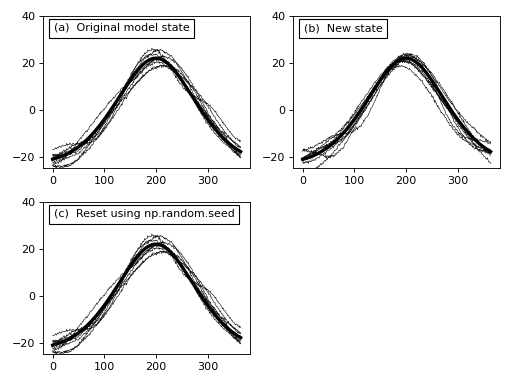# Data sample modeling¶

Consider the Canadian temperature dataset in the figure below. These data are from Ramsay & Silverman (2005) and are available for download here:

The data are in fdaMatlab.zip in the /examples/weather folder.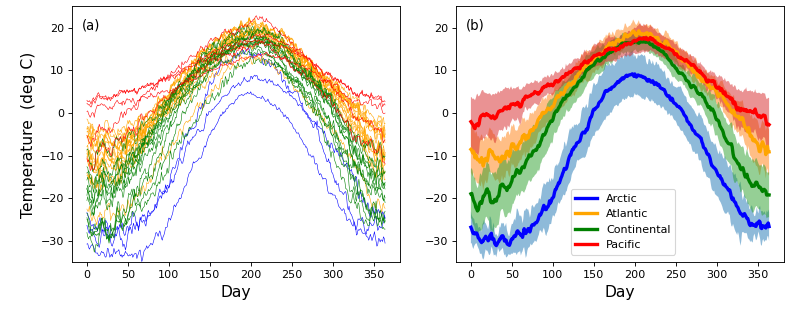The first step of power analysis is to create a model the data that can be used to generate real numerical data samples. Later those data samples will be iteratively generated over a large number of iterations in order to numerically accumulate probability distributions associated with the model.

In power1d there are four steps to creating a data sample model:

1. Create baseline geometry.

2. Create signal geometry.

3. Create a noise model.

4. Combine 1–3 in a DataSample model.

The first step is to create “baseline” one-dimensional (1D) geometry. If one has experimental data like those depicted above, it is easy to generate that baseline as a power1d.geom.Continuum1D as follows:

```import power1d

data     = power1d.data.weather()  # load data
y        = data['Continental']     # extract one region
m        = y.mean( axis=0 )        # mean continuum

baseline = power1d.geom.Continuum1D( m )
baseline.plot()
```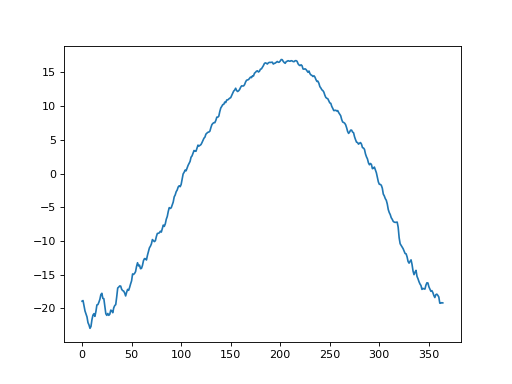If we didn’t have experimental data we could approximate the baseline using the geometric models in power1d.geom. In this case one possibility for modeling the baseline is to subtract a Constant value from a GaussianPulse as indicated below. This appears to create a reasonable approximation to the experimental data.

```from matplotlib import pyplot
import power1d

data     = power1d.data.weather()  # load data
y        = data['Continental']     # extract one region
m        = y.mean( axis=0 )        # mean continuum

# approximate the experimental value using a Gaussian pulse:
Q        = 365  # continuum size
g0       = power1d.geom.GaussianPulse( Q , q=200 , fwhm=190 , amp=40 )
g1       = power1d.geom.Constant( Q , amp=23 )
baseline = g0 - g1  # subtract the geometries

ax       = pyplot.axes()
ax.plot( m , label='Experimental mean', color='k')
baseline.plot( ax=ax, color='g', linewidth=3, label='Model' )
ax.legend()
```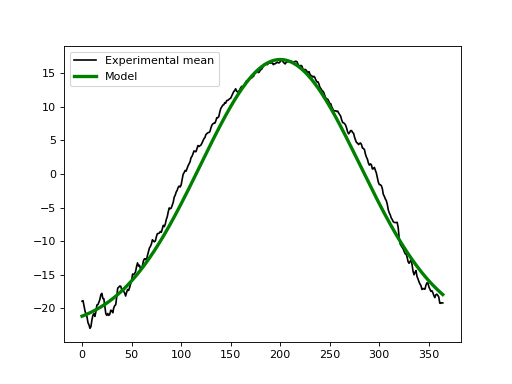The second step is to create a signal that we wish to detect experimentally. Let’s say that we hope to detect a broad increase in temperature over the summer months with a maximum temperature increase of 5 deg. That could be modeled as a second Gaussian pulse like this:

```from matplotlib import pyplot
import power1d

data     = power1d.data.weather()  # load data
y        = data['Continental']     # extract one region
m        = y.mean( axis=0 )        # mean continuum

# approximate the experimental value using a Gaussian pulse:
Q        = 365  # continuum size
g0       = power1d.geom.GaussianPulse( Q , q=200 , fwhm=190 , amp=40 )
g1       = power1d.geom.Constant( Q , amp=23 )
baseline = g0 - g1  # subtract the geometries

signal   = power1d.geom.GaussianPulse( Q , q=200 , fwhm=100 , amp=5 )

ax       = pyplot.axes()
ax.plot( m , label='Experimental mean', color='k')
baseline.plot( ax=ax, color='g', linewidth=3, label='Model' )
(baseline + signal).plot( ax=ax, color='r', linewidth=3, label='Signal' )
ax.legend()
```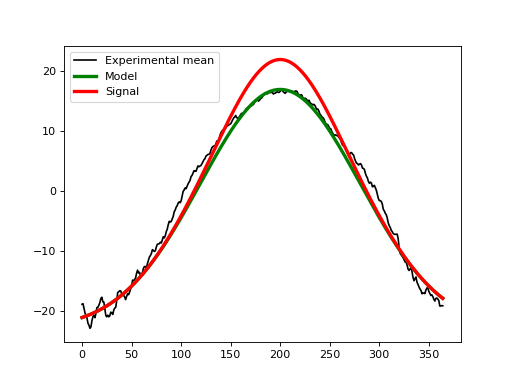The third step is to create a model of the noise we expect to observe experimentally. From the weather data above we can see at least two types of noise: a high-frequency day-to-day noise and lower-frequency noise across the different 1D observations. In power1d a variety of noise models are available to model theses processes. Here let’s model the noise as a combination of uncorrelated Gaussian noise and SmoothGaussian noise:

```from matplotlib import pyplot
import power1d

J        = 8    # sample size
Q        = 365  # continuum size
noise0   = power1d.noise.Gaussian( J , Q , mu = 0 , sigma = 0.3 )
noise1   = power1d.noise.SmoothGaussian( J , Q , mu = 0 , sigma = 3 , fwhm = 70 )
noise    = power1d.noise.Additive( noise0 , noise1 )

ax0      = pyplot.subplot(221)
ax1      = pyplot.subplot(222)
ax2      = pyplot.subplot(223)
AX       = [ax0, ax1, ax2]

# plot noise objects:
noise0.plot( ax=ax0 )
noise1.plot( ax=ax1 )
noise.plot( ax=ax2 )
pyplot.setp(AX, ylim=(-9, 9))

for ax,s in zip( AX , labels ):
ax.text(0.05, 0.9, s, transform=ax.transAxes, bbox=dict(facecolor='w'))
```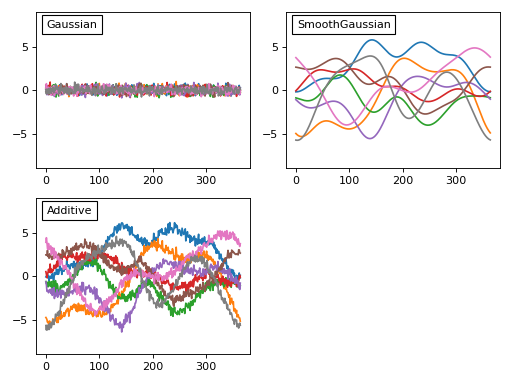Before we add that noise to our model let’s consider these noise models briefly. Each noise model has an attribute value which contains a (J x Q) array representing the current state of the random noise. New random noise can be generated using the random method, and the noise values can be replicated using NumPy’s np.random.seed function as follows:

```from matplotlib import pyplot
import power1d

J = 3
Q = 5

ax0      = pyplot.subplot(221)
ax1      = pyplot.subplot(222)
ax2      = pyplot.subplot(223)
AX       = [ax0, ax1, ax2]

# (a) generate noise object:
np.random.seed(12345)
noise    = power1d.noise.Gaussian(J, Q, mu=0, sigma=1)
noise.plot( ax=ax0 )

# (b) create new random data:
noise.random()
noise.plot( ax=ax1 )

# (c) reset noise to its original state:
np.random.seed(12345)
noise.random()
noise.plot( ax=ax2 )

labels   = '(a)  Original noise', '(b)  New noise', '(c)  Reset using np.random.seed'
for ax,s in zip( AX , labels ):
ax.text(0.05, 0.9, s, transform=ax.transAxes, bbox=dict(facecolor='w'))
```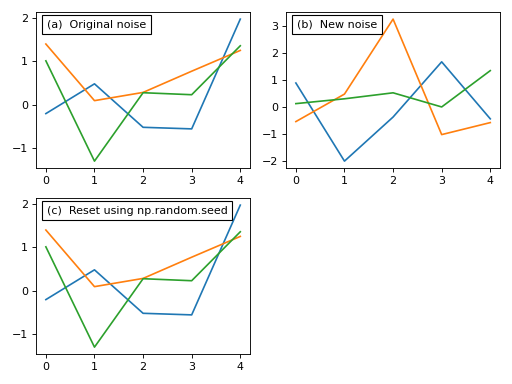This noise control is identical for all types of noise models including compound types like the Additive model above.

The fourth and final step is to combine our baseline, signal and noise objects into a DataSample object like this:

```from matplotlib import pyplot
import power1d

J        = 8    # sample size
Q        = 365  # continuum size

# construct baseline geometry:
g0       = power1d.geom.GaussianPulse( Q , q=200 , fwhm=190 , amp=40 )
g1       = power1d.geom.Constant( Q , amp=23 )
baseline = g0 - g1  # subtract the geometries

# construct signal geometry:
signal   = power1d.geom.GaussianPulse( Q , q=200 , fwhm=100 , amp=5 )

# construct noise model:
noise0   = power1d.noise.Gaussian( J , Q , mu = 0 , sigma = 0.3 )
noise1   = power1d.noise.SmoothGaussian( J , Q , mu = 0 , sigma = 3 , fwhm = 70 )
noise    = power1d.noise.Additive( noise0 , noise1 )

# create data sample model:
model    = power1d.models.DataSample( baseline, signal, noise, J=J )

# visualize
ax0      = pyplot.subplot(221)
ax1      = pyplot.subplot(222)
ax2      = pyplot.subplot(223)
AX       = [ax0, ax1, ax2]

np.random.seed(12345)
model.random()
model.plot( ax=ax0 )

model.random()
model.plot( ax=ax1 )

np.random.seed(12345)
model.random()
model.plot( ax=ax2 )

pyplot.setp(AX, ylim=(-25, 40))